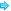科研进展新闻动态科研进展综合新闻传媒扫描
 现在位置：首页 > 新闻动态 > 科研进展

2023-09-25 | 编辑：

A locally optimal preconditioned Newton-Schur method is proposed for solving symmetric elliptic eigenvalue problems. Firstly, the Steklov-Poincaré operator is used to project the eigenvalue problem on the domain \Omega onto the nonlinear eigenvalue subproblem on \Gamma, which is the union of subdomain boundaries. Then, the direction of correction is obtained via applying a non-overlapping domain decomposition method on \Gamma. Four different strategies are proposed to build the hierarchical subspace U_{k+1} over the boundaries, which are based on the combination of the coarse-subspace with the directions of correction. Finally, the approximation of eigenpair is updated by solving a local optimization problem on the subspace U_{k+1}. The convergence rate of the locally optimal preconditioned Newton-Schur method is proved to be \Gamma =1-c_{0}T_{h,H}^{-1}, where c_{0} is a constant independent of the fine mesh size h, the coarse mesh size H and jumps of the coefficients; whereas T_{h,H} is the constant depending on stability of the decomposition. Numerical results confirm our theoretical analysis.

Publication:

Mathematics of Computation, 92 (2023), 2655-2684

Author:

Wenbin Chen

School of Mathematical Sciences and Shanghai Key Laboratory for Contemporary Applied Mathematics, Fudan University, Shanghai 200433, People’s Republic of China

Nian Shao

School of Mathematical Sciences, Fudan University, Shanghai 200433, People’s Republic of China

Xuejun Xu

LSEC, Institute of Computational Mathematics, Academy of Mathematics and System Sciences, Chinese Academy of Sciences, Beijing 100190, People’s Republic of China

Email: xxj@lsec.cc.ac.cn

【打印本页】【关闭本页】